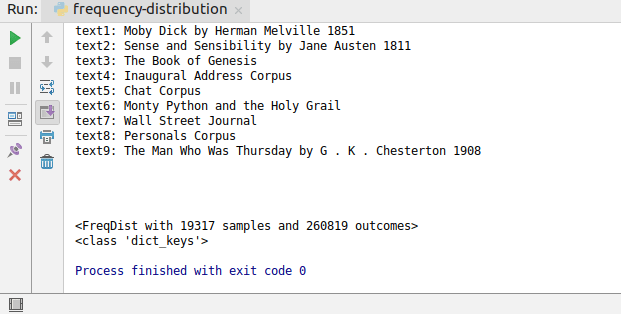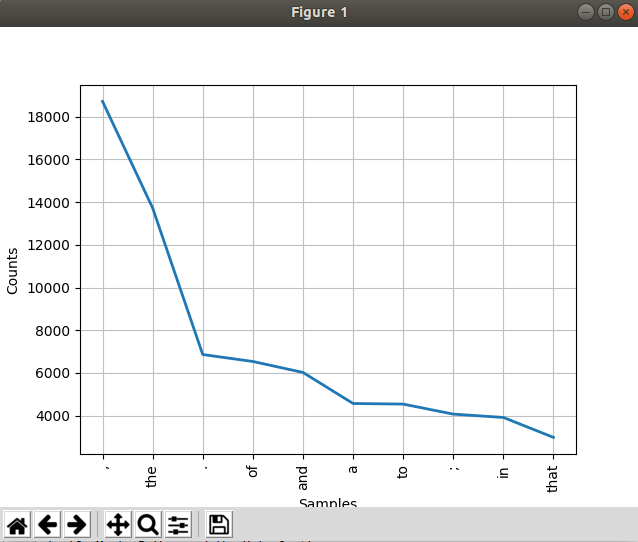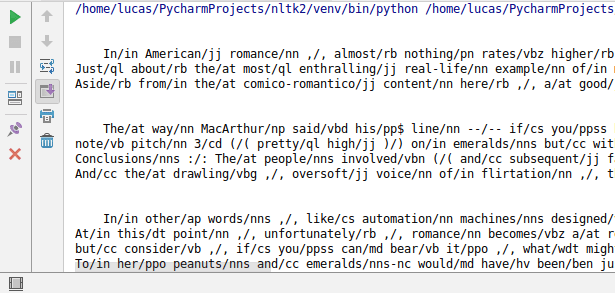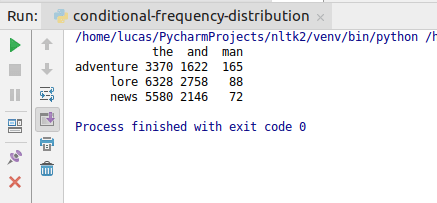# Frequency Distribution in NLTK

After learning about the basics of Text class, you will learn about what is Frequency Distribution and what resources the NLTK library offers.

Tutorial Contents

## Frequency Distribution

So what is frequency distribution? This is basically counting words in your text. To give you an example of how this works, create a new file called frequency-distribution.py , type following commands and execute your code:The class FreqDist  works like a dictionary where the keys are the words in the text and the values are the count associated with that word. For example, if you want to see how many words “man” are in the text, you can type:

One important function in FreqDist  class is the .keys()  function. To see what it does, type in your code:

So if you run your code now, you can see that it returns you the class dict_keys , in other words, you get a list of all the words in your text.To see how many words there are in the text, you can say:

From the previous tutorial, you can remember that the class nltk.text.Text  has functions that do the same stuff. So what is the difference? The difference is that with FreqDist  you can create your own texts without the necessity of converting your text to nltk.text.Text  class.

Another useful function is plot . What plot does is it displays the most used words in the text. So if you want the ten most used words in the text, for example, you can type:

and you will get a graph like in the image below:## Personal Frequency Distribution

So let’s say that you want to do a frequency distribution based on your own personal text. To do this, create a new file named personal-frequency-distribution.py  and type the following code:

Let’s go throughout our code now.

As you can see in the first line, you do not need to import nltk.book  to use the FreqDist  class. So if you do not want to import all the books from nltk.book  module, you can simply import FreqDist  from nltk.

We then declare the variables text  and text_list . The variable text  is your custom text and the variable text_list  is a list that contains all the words of your custom text. You can see that we used text.split(" ")  to separate the words.

Then you have the variables freqDist  and words. freqDist  is an object of the FreqDist  class for your text and words is the list of all keys of freqDist .

The last line of code is where you print your results. In this example, your code will print the count of the word “free”.

If you replace “free” with “you”, you can see that it will return 1 instead of 2. This is because nltk indexing is case-sensitive. To avoid this, you can use the .lower() function in the variable text.

## Conditional Frequency Distribution

Now you know how to make a frequency distribution, but what if you want to divide these words into categories? For this, you have another class in nltk  module, the ConditionalFreqDist .

To give you an example of how this works, import the Brow corpus with the following line:

If you say

you can see that this corpus is divided into categories. Each of these categories contains some textual data that can be accessed through the following command:

Pay attention to the categories  keyword. In this parameter, we pass a string that contains the name of the category we want. You can use the raw function in a similar way:If you see the output, you will notice that the words have a / . It will be covered in a later tutorial but for now, we can say that each textual data is mapped for analysis.

Moving forward to what conditional frequency distribution is, we can say that if your text is divided into categories, you can maintain separate frequency distributions for each category. In our example, our corpus has categories like adventure, editorial, fiction, etc.

The first thing you need to do is import the conditional frequency distribution class which is located in the nltk  module directly. In your code, type the import statement:

The ConditionalFreqDist  class expects a list of tuples in its constructor. The first value of the tuple is the condition and the second value is the word. You can create a list where the condition is the category where the word is with the following line of code:

You can have a better idea of what is going on if you print the contents of the pair_list variable. So add the following line:

As you can see, the list contains tuples where the first element is the category and the second element is a word in that category.

Now, you can create a ConditionalFreqDist  object. In your code write

As you can see, your ConditionalFreqDist  object has 15 conditions because the Brown Corpus contains 15 categories but what can you do with it? Let’s say you want to see how many times the word “the” occur in the category “lore”, you can do it with the following line:

If you want to know the conditions that are being applied in your conditional frequency distribution, you can use the conditions function:

Now, a useful function you should pay attention is the tabulate  function. With that function, you can count how many times a given word occurs in certain categories and display it in a tabular format.

To give you an example on how this works, let’s say you want to know how many times the words “the”, “and” and “man” appear in “adventure”, “lore” and “news”.

So the tabulate  function expects two parameters, the category, and the samples. In your case, the categories are “adventure”, “lore” and “news” while your samples are “the”, “and” and “man”.

If you run this code, you can see that it returns you the data presented in a table.This is all for the tutorial. If you have any question, feel free to leave it in the comments below.

## NLTK Course

Join our NLTK comprehensive course and learn how to create sophisticated applications using NLTK, including Gender Predictor, and Document Classifier, Spelling Checker, Plagiarism Detector, and Translation Memory system.

https://www.udemy.com/natural-language-processing-python-nltk/?couponCode=NLTK-BLOGS